# Negative time difference of simultaneous events?

chipotleaway
If two events separated in space by Δx occur simultaneously according to an observer S, then the time difference for S' moving at velocity u relative to S is

$$\Delta t' = \frac{-u\Delta x}{c^2\sqrt{1-\frac{u^2}{c^2}}}$$

Why is Δt' negative?

$\Delta t' = t'_2 - t'_1$, where $t'_2$ corresponds to time of event 2 and likewise for $t'_1$ and event 1.
At 40:45 in this lecture, he says that this is because the event with the greater coordinate occurs later. But wouldn't this mean that $t'_2>t'_1$ and so Δt' should be positive?

*

Also, is the following statement correct?
When we say such and such events occur at some place and time for S, we have taken into account all relativistic effects, so the events are not necessarily at rest for S (so in original question, S would be travelling at some velocity such that the events are simultaneous, seperated by Δx). Therefore when we want to describe the events as seen by S', the velocity u of S' we use is relative to S, and we can only say that it's relative to both S and the events if S is at rest relative to the events.

Thanks

Last edited by a moderator:

## Answers and Replies

yuiop
If two events separated in space by Δx occur simultaneously according to an observer S, then the time difference for S' moving at velocity u relative to S is

$$\Delta t' = \frac{-u\Delta x}{c^2\sqrt{1-\frac{u^2}{c^2}}}$$

Why is Δt' negative?

If the spatially separated events in S are simultaneous, then they are spacelike separated and so are not causally linked. This means the order of these events is relative and we can find refrence frames where they occur in any order we like. For example if ##\Delta x## or u is negative then ##\Delta t'## would be positive.

$\Delta t' = t'_2 - t'_1$, where $t'_2$ corresponds to time of event 2 and likewise for $t'_1$ and event 1.
At 40:45 in this lecture, he says that this is because the event with the greater coordinate occurs later. But wouldn't this mean that $t'_2>t'_1$ and so Δt' should be positive?
I assume here he is talking about timelike separated events so they are causally linked and then if $t'_2>t'_1$ is true in one reference frame then it is true in all reference frames.

chipotleaway
When would $\Delta x$ be negative? If two events are simultaneous according to S, then which event is considered first and second? So then how is $\Delta x$ defined - the larger event with the larger x-coordinate minus the smaller?

So assuming it is, what if $\Delta x$ and u are both positive (as in the case I'm thinking of) $x_1$ is at a lesser distance from the origin of Sthan $x_2$ and S' is moving to the right at speed u so is positive.

If $\Delta t'$ is negative, then this implies that the event at $x_1$ occurs after the event at $x_2$?

yuiop
When would $\Delta x$ be negative? If two events are simultaneous according to S, then which event is considered first and second? So then how is $\Delta x$ defined - the larger event with the larger x-coordinate minus the smaller?
Good question. I certainly had to pause for thought. I think for two simultaneous events most people would treat it as a magnitude rather than a vector and simply use the method you suggest. However, I do not think there is any hard an fast rule about this and if the events are not simultaneous, I think most people would assign coordinates such that ##\Delta t## is positive and ##\Delta x## takes on whatever sign is necessary. Consider two events A and B that are almost simultaneous such that t2 = 0 and t1 = dt where A always has the lesser x coordinate. If the t coordinate of A is 0, then we might assign coordinates (x1,t1) to event A and (x2,t2) to coordinate B. Since dt is chosen to be negligible compared to the values of u and '##\Delta X##, the value of ##\Delta t'## is negative. Now if the t coordinate of A is dt, then we might assign coordinates (x2,t2) to event A and (x1,t1) to coordinate B and the value of ##\Delta t'## is now positive. This tiny change of dt brings about a complete sign reversal of ##\Delta t'## but you will see that is not anything to worry about. This is because all we have done is relabel the events. A coordinete independent way of comparing the separtion of events A and B is to use the spacetime interval ##dS^2##:

##dS^2 = \Delta x^2 - \Delta t^2##

It is obvious from the above that ##dS^2## is not affected by the sign of ##\Delta t##.

So assuming it is, what if $\Delta x$ and u are both positive (as in the case I'm thinking of) $x_1$ is at a lesser distance from the origin of S than $x_2$ and S' is moving to the right at speed u so is positive.

If $\Delta t'$ is negative, then this implies that the event at $x_1$ occurs after the event at $x_2$?
That all depends on which reference frame you are referring to. $x_1$ and $x_2$ are coordinates in S and the event $x_1$ certainly does not come after (or before) the event at $x_2$ as they are defined to be simultaneous in that frame. You are right that in frame S' that $x_1'$ occurs after the event at $x_2'$, but there are other reference frames, e.g. those moving with velocity -u relative to S where $x_1'$ occurs before the event at $x_2'$,

•1 person
Gold Member
If two events separated in space by Δx occur simultaneously according to an observer S, then the time difference for S' moving at velocity u relative to S is

$$\Delta t' = \frac{-u\Delta x}{c^2\sqrt{1-\frac{u^2}{c^2}}}$$

Why is Δt' negative?

$\Delta t' = t'_2 - t'_1$, where $t'_2$ corresponds to time of event 2 and likewise for $t'_1$ and event 1.
At 40:45 in this lecture, he says that this is because the event with the greater coordinate occurs later. But wouldn't this mean that $t'_2>t'_1$ and so Δt' should be positive?

*

I didn't watch the whole video but I did watch a few minutes prior to your quote and the professor doesn't define which event is 1 or 2. It doesn't matter but you have to be consistent. In his example, he talks about a train car in which two events are generated by a light beams emitted at the center of the car and striking the two ends. Then he considers the train car to be moving to the right and he discusses what an observer on the ground would determine for the delta time between the events. Here is a spacetime diagram depicting the train car in its rest frame. The observer in the middle of the train car is shown in blue and the observer on the ground is show in green. I'm using units where the value of c=1 so I can drop it out of the equations: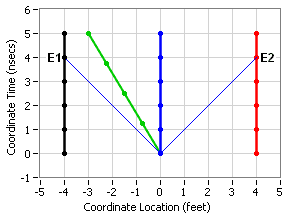The coordinates for event 1 (E1) are:

t1=4, x1=-4
t2=4, x2=4

Therefore, following your preference for ordering the events:

Δx=x2-x1
Δx=4-(-4)
Δx=4+4
Δx=8

Since we want to see what Δt' is for the green ground observer, we note that his speed, u, in the S frame is -0.6c. So applying the formula:

Δt'=-uΔx/√(1-u2)
Δt'=-(-0.6)(8)/√(1-(-0.6)2)
Δt'=4.8/√(1-0.36)
Δt'=4.8/√0.64
Δt'=4.8/0.8
Δt'=6

Now to confirm this, we can transform the above diagram into one for the rest frame of the green ground observer: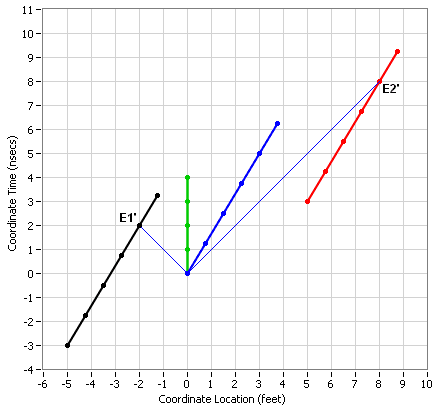Sure enough, the primed events are:

t1'=2, x1'=-2
t2'=8, x2'=8

Δt'=t2'-t1'
Δt'=8-2
Δt'=6

You could have interchanged the assignment of the labels for the two events, in which case Δt' would come out to be a negative number, just as it should.

You must have been thinking that u is positive but I hope you can see in the professor's example it is negative.

Also, is the following statement correct?

When we say such and such events occur at some place and time for S, we have taken into account all relativistic effects, so the events are not necessarily at rest for S (so in original question, S would be travelling at some velocity such that the events are simultaneous, seperated by Δx). Therefore when we want to describe the events as seen by S', the velocity u of S' we use is relative to S, and we can only say that it's relative to both S and the events if S is at rest relative to the events.

Thanks
No, that statement keeps talking about the events in motion or at rest, but those attributes don't apply to events which are instants in time at specific locations. You should think of frame S' (or an observer at rest in S') moving with respect to frame S and determine which direction that is when deciding on the sign of u.

#### Attachments

Last edited by a moderator:
•1 person
phyti
If two events separated in space by Δx occur simultaneously according to an observer S, then the time difference for S' moving at velocity u relative to S is

$$\Delta t' = \frac{-u\Delta x}{c^2\sqrt{1-\frac{u^2}{c^2}}}$$

Why is Δt' negative?

Thanks

Here is a graphic showing the possible order of events, depending on direction.

https://www.physicsforums.com/attachments/62723

Last edited:
•1 person
chipotleaway
Sorry for late response, was focused on assignments based on other topics and subjects so didn't have much time to think about this.

Anyway, the negative time difference for the train example makes sense now (got it from the algebra, still having a bit of trouble interpreting spacetime diagrams) , but what if it was S' that was moving to the right relatve to S? Then the velocity of S' relative to S would be positive and so the time difference would be negative.

Gold Member
...Anyway, the negative time difference for the train example makes sense now (got it from the algebra, still having a bit of trouble interpreting spacetime diagrams)
What sort of trouble are you having? I always try to make my spacetime diagrams as easily understandable as possible and if they're not, I'd like to find out why so that I can improve on them.

but what if it was S' that was moving to the right relatve to S? Then the velocity of S' relative to S would be positive and so the time difference would be negative.

Yes, as long as you continue to maintain the same assignment of labels. Here are a couple of diagrams depicting your new scenario: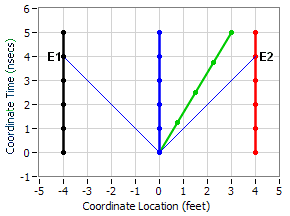And: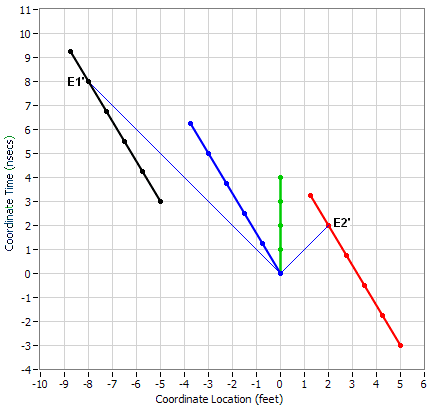But you could even more easily just interchange the labels and gotten a delta with a negative value.

#### Attachments

•1 person
chipotleaway
No, the diagrams as clear as they can be! The fault is on my part - I am rather slow

That's kind of unintuitive then (though I don't recall ever hearing anyone say that relativity was)...the event further away occurs first..

Gold Member
the event further away occurs first..
But in your first post you said:
the event with the greater coordinate occurs later.

which sounds the opposite of what you are saying now.

I don't think either saying is correct because the order of events depends on the selected Inertial Reference Frame (IRF), not on how far away they are (whatever that means). In one IRF (the first diagram in post #5 or #8), E1 and E2 occur at the same time. In another IRF (the second diagram in post #5) E1 occurs first, while in a third IRF (the second diagram in post #8) E2 occurs first.

Let me show you another example. We start with two simultaneous events in this IRF: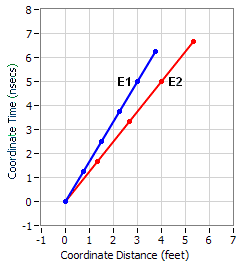Transforming to an IRF moving at -0.3c with respect to the first IRF, we see that E1 occurs first: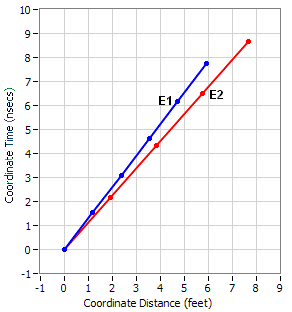But transforming to an IRF moving at 0.3c with respect to the first IRF, we see that E2 occurs first: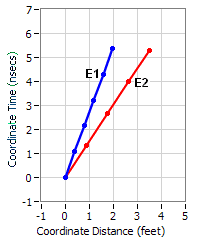So I would say that following your intuition or pithy sayings is dangerous. Just use the Lorentz Transformation process and you'll never go wrong.

#### Attachments

•1 person
chipotleaway
chipotleaway said:
the event with the greater coordinate occurs later.

Here, that's what Prof. Shankar said and I thought that should imply that the time difference be positive, not negative.
This is for when S is moving away from S' (increasing x on the x-axis) and from S point of view, S' has negative velocity which from your post, the Δt' turns out to be positive.

chipotleaway said:
the event further away occurs first..

Here, S' is moving towards S from the left of it on the x-axis and from S point of view, S' has positive velocity, which makes Δt' negative, implying that the event with the bigger x-coordinate occurs first.

#### Attachments

Gold Member
the event with the greater coordinate occurs later.
Here, that's what Prof. Shankar said and I thought that should imply that the time difference be positive, not negative.
This is for when S is moving away from S' (increasing x on the x-axis) and from S point of view, S' has negative velocity which from your post, the Δt' turns out to be positive.
The professor's statement is only true when the velocity is negative.

By the way, I watched more of the video and he does specify the order of the events at 24:27, namely that Δx = x2-x1.
the event further away occurs first..

Here, S' is moving towards S from the left of it on the x-axis and from S point of view, S' has positive velocity, which makes Δt' negative, implying that the event with the bigger x-coordinate occurs first.
This statement is only true when the velocity is positive.

But his equation from your first post is always true with his definition of the order of the events.

Are you clear on everything or do you have more questions?

•1 person
chipotleaway
This statement is only true when the velocity is positive.

But his equation from your first post is always true with his definition of the order of the events.

Are you clear on everything or do you have more questions?

Ya this was the (more) 'unintuitive' part for me. I think that's all for now, should tackle some more problems to get my head around this.

Thanks for continuing to reply and sorry about the delay between my posts.